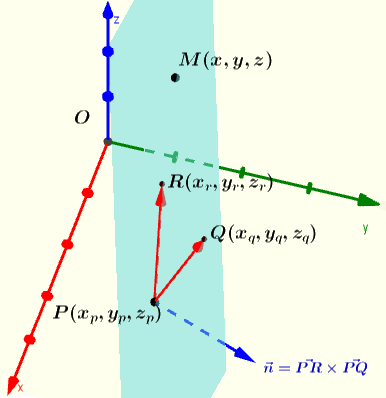# Equation of a Plane Through three Points


 An interactive worksheet including a calculator and solver to find the equation of a plane through three points is presented. As many examples as needed may be generated interactively along with their solutions and explanations. Below is shown a plane passing through the three points $$P(x_p,y_p,z_p)$$, $$Q(x_q,y_q,z_q)$$ and $$R(x_r,y_r,z_r)$$.We first define vector $$\vec {n}$$ as the cross product of vectors $$\vec {PR}$$ and $$\vec {PQ}$$ $$\vec {n} = \vec {PR} \times \vec {PQ}$$ From definition of the cross product, $$\vec {n}$$ is perpendicular to both vectors $$\vec {PR}$$ and $$\vec {PQ}$$ and therefore to the plane containing all three points P, Q and R. Any point $$M(x,y,z)$$ is on the plane if the dot product of $$\vec n = \lt x_n,y_n,z_n \gt$$ and vectors $$\vec {PM} = \lt x - x_p , y - y_p , z - z_p \gt$$ is equal to zero. $$\vec n \cdot \vec {PM} = ( \vec {PR} \times \vec {PQ}) \cdot \vec {PM} = \lt x_n,y_n,z_n \gt \cdot \lt x - x_p , y - y_p , z - z_p \gt = 0$$ Step by step solution STEP 1: Find vectors PR and PQ and calculate vector n = PR x PQ. STEP 2: Write the components of vector PM. STEP 3: Write that the dot product of vectors n and PM is equal to zero. STEP 4: Expand the product, simplify and write the equation in the form a x + b y + c z = d.
More Step by Step Math Worksheets Solvers New !